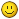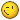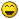# PnCConf spindle max rpm limit issue

25 Mar 2020 06:11 #161394 by Dex64
Hello

I have 5i25 and 7i77 cards. Now I try to setting spindle in pncconf. My spindle has 24000rpm. How can I add this spindle rpm to configuration ? The limit in pncconf is 10000rpm ( 0-10V). When I change 10k rpm to 24k rpm manually in .ini file it doesn't work.
See attachment##### Attachments:

25 Mar 2020 07:24 #161395 by cmorley
What version of linuxcnc are you using?
You will have to stick with 10000 and then edit the INI file after.

In the INI file you'll see something like this at the bottom:
```[SPINDLE_0]
P = 0.0
I = 0.0
D = 0.0
FF0 = 1.0
FF1 = 0.0
FF2 = 0.0
BIAS = 0.0
MAX_OUTPUT = 10000.0
OUTPUT_SCALE = 10000
OUTPUT_MIN_LIMIT = -10000
OUTPUT_MAX_LIMIT = 10000
```

Change all (four) of the 10000 to 24000, keep the signs the same as your file.

Chris

25 Mar 2020 07:40 #161396 by cmorley

25 Mar 2020 07:45 #161397 by Dex64
I will check it soon. Thanks for answer25 Mar 2020 07:46 #161398 by cmorley
I just noticed you didn't select +-10 volt output so this would be they way it should be:
```[SPINDLE_0]
P = 0.0
I = 0.0
D = 0.0
FF0 = 1.0
FF1 = 0.0
FF2 = 0.0
BIAS = 0.0
MAX_OUTPUT = 24000.0
OUTPUT_SCALE = 24000
OUTPUT_MIN_LIMIT = 0
OUTPUT_MAX_LIMIT = 24000
```

I'll increase the limit to 100 000 in the future
Chris

25 Mar 2020 16:13 #161469 by Dex64
I have version 2.7.0. I tried to put settings as You said but it didn't work. Work propertly with settings:

[SPINDLE_9]
P = 0.0
I = 0.0
D = 0.0
FF0 = 1.0
FF1 = 0.0
FF2 = 0.0
BIAS = 0.0
MAX_OUTPUT = 6000.0
OUTPUT_SCALE = 1.0
OUTPUT_MIN_LIMIT = 0
OUTPUT_MAX_LIMIT = 1

When I try to change MAX_OUTPUT = 24000.0 then Linux see still this 6000rpm.
When I set M3 S1000, then spindle has 4000rpm etc....10V at analogout5 is when I set S6000 or more.
I'm beginner in linuxcncThanks for understanding.

25 Mar 2020 20:10 #161498 by cmorley
Well I can;t see how that works at all.
Can you post your config files?

26 Mar 2020 02:27 #161531
I have a Huanyang 24K spindle and these are my ini settings. I can't remember where I got the 16800 number from but I can run the spindle up to 400hz 24000 rpm.

[SPINDLE_0]
P = 0
I = 0
D = 0.0
FF0 = 1.00
FF1 = 0.0
FF2 = 0.0
BIAS = 0.0
OUTPUT_MIN_LIMIT = 0
OUTPUT_MAX_LIMIT = 16800
OUTPUT_SCALE = 16800

26 Mar 2020 20:46 #161616 by Dex64
Here are my config files. Ps. Don't worry about PID settings in XYZ axes I will set it later.